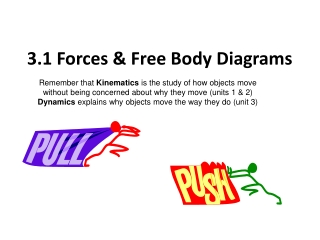DownloadDownload Presentation3.1 Forces & Free Body Diagrams

# 3.1 Forces & Free Body Diagrams

Télécharger la présentation## 3.1 Forces & Free Body Diagrams

- - - - - - - - - - - - - - - - - - - - - - - - - - - E N D - - - - - - - - - - - - - - - - - - - - - - - - - - -
##### Presentation Transcript

1. 3.1 Forces & Free Body Diagrams Remember that Kinematics is the study of how objects move without being concerned about why they move (units 1 & 2) Dynamics explains why objects move the way they do (unit 3)

2. What forces are acting on the ice cream cart?

3. Force • A push or a pull • Can make things move or slow them down • Can distort things • stretching, compressing, twisting • Two types: • action-at-a-distance • contact • A vector quantity

4. Fundamental Forces • Gravitational force • Force of attraction between all objects in the universe • Electromagnetic force • Caused by electric charges • Holds atoms and molecules together • Strong nuclear force • Holds nucleus of an atom together • Weak nuclear force • Responsible for elementary particle interactions • Only effective over small distances

5. Units of Force: • Measured/calculated in Newtons • 1 N represents the amount of force required to accelerate a mass of 1 kg at a rate of 1 m/s2. 1 N = 1 kg m s2

6. Some Common Forces:

7. Force Due to Gravity • Where and mass is in kg • Ex: What is the force of gravity of a person weighing 60kg?

8. Force Diagrams To analyze forces and their effects, we use two types of diagrams: System diagram: includes all objects under analysis (eg a drawing of a book sitting on a desk) Free-body diagram (FBD): shows all forces acting on the object being analyzed How to draw FBDs: • Object can be represented by a square/rectangle/circle • Forces are represented by vectors (arrows) • Lengths of vectors are not to scale but are proportional to magnitudes of forces • Indicate which direction is positive

9. An Example:

10. Another example: FN Ff FA Fg

11. For practise (right now!): Try drawing the diagrams for

12. Solutions

13. Calculating Net Forces • You must combine all the forces acting on the object as a single combined force. Ex1: A stationary object exerts a force of gravity of 45N [down] and the floor exerts a normal force of 45 N [up]. What is the next force? =45N = = =-45N Therefore there is no net force on the stationary object and therefore it does not move in any direction

14. Ex2: Calculate the next force of the object = = Therefore the object has a net force of 15N [right] which would cause the object to move to the right Homework: pg 120 # 1-3 pg 122 # 1,2,5,7, 15